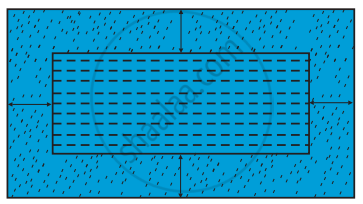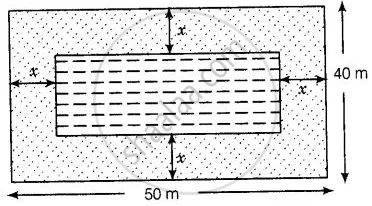# In the centre of a rectangular lawn of dimensions 50 m × 40 m, a rectangular pond has to be constructed so that the area of the grass surrounding the pond would be 1184 m2 - Mathematics

Sum

In the centre of a rectangular lawn of dimensions 50 m × 40 m, a rectangular pond has to be constructed so that the area of the grass surrounding the pond would be 1184 m2 [see figure]. Find the length and breadth of the pond.#### Solution

Given that a rectangular pond has to be constructed in the centre of a rectangular lawn of dimensions 50 mx 40 m

So, the distance between pond and lawn would be same around the pond.

Say x m.Now, length of rectangular lawn (l1) = 50 m and breadth of rectangular lawn (b1) = 40 m

Length of rectangular pond (l2)= 50 – (x + x) = 50 – 2x

And breadth of rectangular pond (b2) = 40 – (x + x)= 40 – 2x

Also, area of the grass surrounding the pond = 1184 m2

Area of rectangular lawn – Area of rectangular pond = Area of grass surrounding the pond

l_1 xx b_1 - l_2 xx b_2 = 1184   ......[∵ Area of rectangle = length × breadth]

⇒ 50 xx 40 - (50 - 2x)(40 - 2x) = 1184

⇒ 2000 - (2000 - 80x - 100x + 4x^2) = 1184

⇒ 80x + 100x - 4x^2 = 1184

⇒ 4x^2 - 180x + 1184 = 0

⇒ x^2 - 45x + 296 = 0   ....[By splitting the middle term]

⇒ x^2 - 37x - 8(x - 37) = 0

⇒ x(x - 37) - 8(x - 37) = 0

⇒ (x - 37)(x - 8) = 0

∴ x = 8

At x = 37,

Length and Breadth of pond are – 24 and – 34, respectively but length and

So, x = 37 cannot be possible

∴ Length of pond = 50 – 2x

= 50 – 2(8)

= 50 – 16

= 34 m

And breadth of pond = 40 – 2x

= 40 – 2(8)

= 40 – 16

= 24 m

Hence, required length and breadth of pond are 34 m and 24 m, respectively.

Concept: Nature of Roots
Is there an error in this question or solution?

#### APPEARS IN

NCERT Mathematics Exemplar Class 10